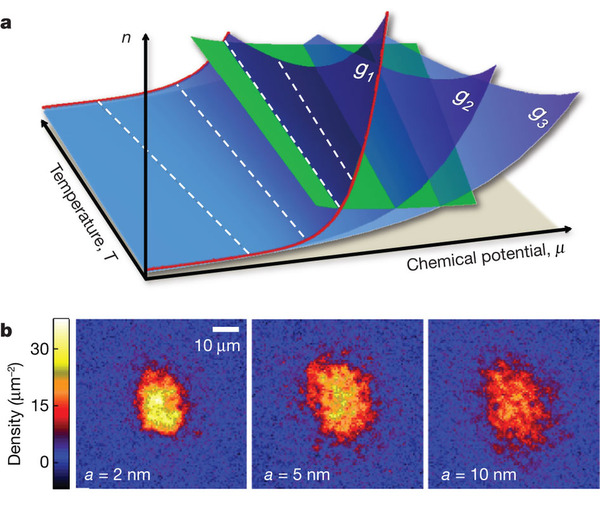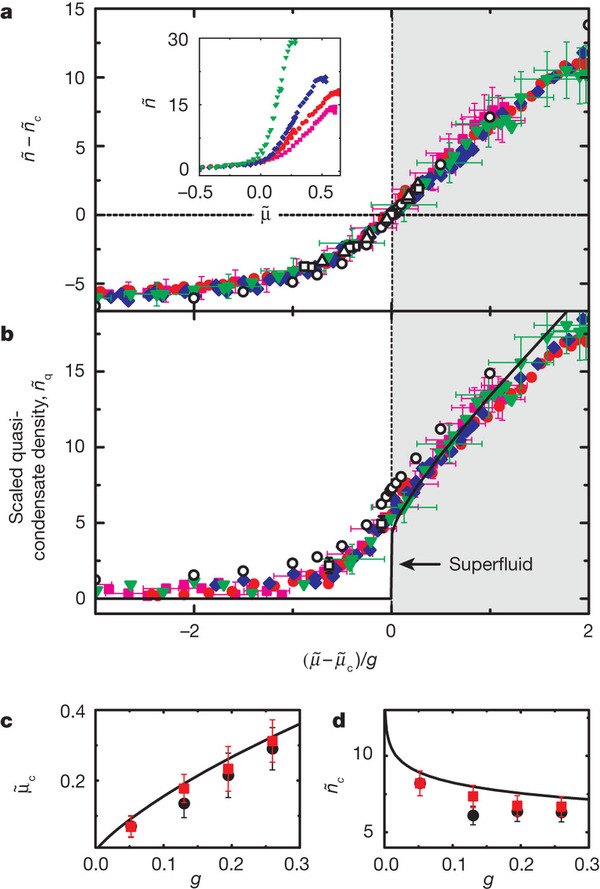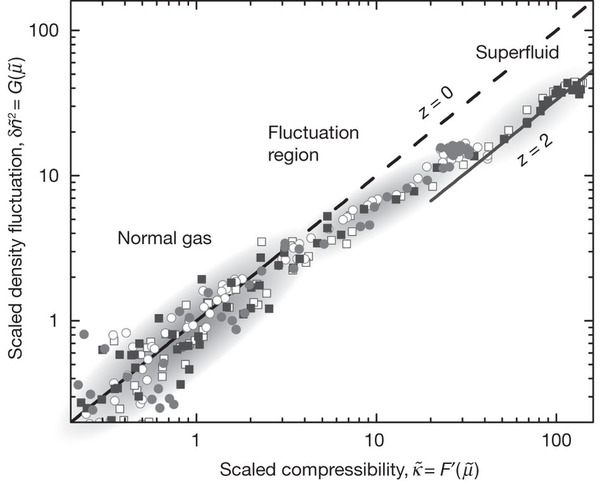# 2011 Cs Experiment

Research show case on the physics department webpage: In situ imaging reveals scaling symmetry in reduced dimensions.

Critical phenomenon near a phase boundary, where the collective behavior of many-body system is insensitive to its microscopic physics and thermodynamic observables follow generalized scaling laws. These scaling behaviors can be categorized into classes determined by the dimensionality and the order of the quantum matter undergoing a continuous phase transitions. One remarkable application of such critical universality, is that a single measurement of a sample prepared under one particular experimental condition can reveal the full information of a many-body system over a wide parameter space. We are currently developing experimental strategies to study critical universal regimes with an aim that our method may be applied to general many-body atomic systems to determine the phase boundaries and diagrams.

In one of our recent work , a major step toward this objective, we investigated the universal scaling property in two-dimensional gases undergoing the Berezinskii-Kosterlitz-Thouless superfluid phase transition . This work describes the first experimental effort to compare in situ images of 2D quantum gases at different temperatures, inter-particle interaction strengths, and chemical potentials, and confirm that many observables develop a universal behavior near a continuous phase transition. We observed in total four universal scaling laws; Through these universal functions, we identified two general methods for determining the phase transition boundary, both showing good agreement between the experimental and the theoretical predictions by classical-field and quantum Monte Carlo calculations. The analysis performed in this work formed an important basis for our objective of studying quantum criticality of 2D gases in an optical lattice.

 Observation of scale invariance and universality in two-dimensional Bose gases

Chen-Lung Hung, Xibo Zhang, Nathan Gemelke, Cheng Chin

Here is a glance through the paper:

###a, Scale invariance links any thermodynamic observable at different chemical potential μ and temperature T via a simple power-law scaling. In a 2D Bose gas with coupling constant g, atomic density n measured at different temperatures (red lines) can be scaled through constant μ/T and n/T contours (dashed lines). Near the BKT phase transition boundary (green plane), systems with different g = g1, g2, ... (blue planes) scale universally. b, In situ density measurements of trapped 2D gases provide crucial information to test the hypotheses of scale invariance and universality. Sample images at different scattering lengths a are obtained from single shots.

###Knowing exactly the trap potential energy at each point of a trapped gas, we can convert the measured density image into the equation of state n(μ,T). We further scale the density into the phase space density, and plot them as a function of the scaled chemical potential μ/T to test the scale invariant hypothesis. What are shown in a are measurements at five different temperatures: T = 21 nK (filled black circles), 37 nK (red squares), 42 nK (green triangles), 49 nK (blue diamonds) and 60 nK (magenta stars), and coupling strength g = 0.26. Mean-field expectations for normal gas (dashed line) and superfluid (solid line) are shown for comparison. Inset shows the radial density profiles before scaling. b, Scaled fluctuation at different temperatures. Dashed line is the mean-field calculation based on the fluctuation-dissipation theorem25. Solid line is an empirical fit to the crossover feature from which the critical chemical potential is determined. Inset, radial fluctuation profiles before scaling. The shaded area marks the fluctuation region.

###a, We proceed on searching for the universal behavior of scaled density profiles measured at various coupling strengths, g = 0.05 (filled green triangles), 0.13 (blue diamonds), 0.19 (red circles) and 0.26 (magenta squares). The collapsing of all equations of state at different g into a single universal curve (details see ) confirms the universality near the BKT critical point. Inset shows the original equations of state. In b, we also confirm the universality of another observable called scaled quasi-condensate density (details see  ). In both plots, Monte Carlo calculations from ref. 12 in  (open circles) and ref. 13 in  (a, open squares for g = 0.07 and open triangles for g = 0.14; b, open squares) are plotted for comparison. The shaded area marks the superfluid regime and the solid line in b shows the superfluid phase space density calculation. In cd, we show the critical density and chemical potential determined from the following methods: universal scaling as shown in a (see ; red squares), density fluctuation crossover (see ; black circles), and Monte Carlo calculations from ref. 11 in  (solid line). Experiment values coincide at g = 0.05 identically, as a result of our analysis (see ).

Last, we conclude the paper with an exciting finding that a growing long-ranged density correlation, similar to the phenomenon that leads to critical opalescence, is revealed near the phase transition:

###Scaled compressibility and scaled density fluctuation are derived from measurements at two interaction strengths, g = 0.05 (squares) and g = 0.26 (circles), each containing two different temperatures between 20 and 40 nK (filled and open symbols, respectively). Diagonal line shows an expectation of G = F′, derived from the fluctuation dissipation theorem. Solid line marks the suppressed local density fluctuation in the superfluid region, hinting the growing density-density correlation in this region. The grey shaded areas mark the normal (left), fluctuation (middle) and superfluid (right) regimes.

For detailed analysis on the density fluctuations and density-density correlations, read our recent paper:

Extracting density-density correlations from in situ images of atomic quantum gases

<IOPSELECT>/<INVITED PAPER IN THEFOCUS ISSUE ON QUANTUM SIMULATION>

Chen-Lung Hung, Xibo Zhang, Li-Chung Ha, Shih-Kuang Tung, Nathan Gemelke, Cheng Chin

New Journal of Physics 13 075019 (2011)